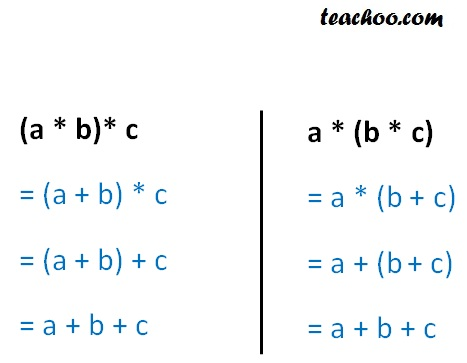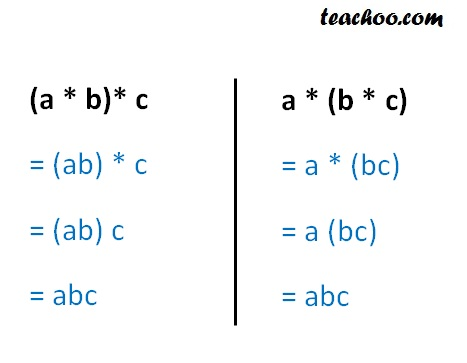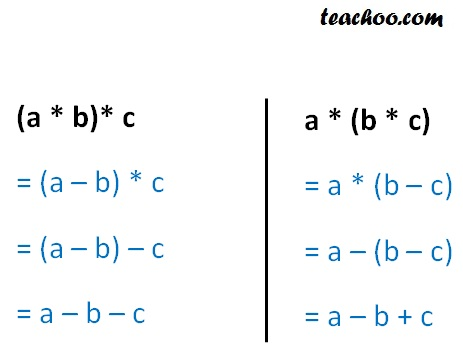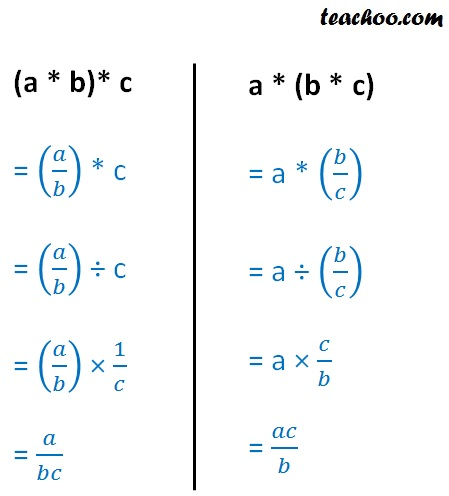Whether binary commutative/associative or not

Chapter 1 Class 12 Relation and Functions
Concept wise

For binary operation

* : A × A → A

If (a * b) * c = a * (b * c)

Then it is associative binary operation

+ : R × R R

* is associative if

(a * b) * c = a * (b * c)Since (a * b) * c = a * (b * c) ∀ a, b, c ∈ R

+ is an associative binary operation

## Multiplication

× : R × R R

* is associative if

(a * b) * c = a * (b * c)Since (a * b) * c = a * (b * c) ∀ a, b, c ∈ R

× is an associative binary operation

## Subtraction

– : R × R R

* is associative if

(a * b) * c = a * (b * c)Since (a * b) * c ≠ a * (b * c) ∀ a, b, c ∈ R

–  is not an associative binary operation

## Division

÷ : R × R R

* is associative if

(a * b) * c = a * (b * c)Since (a * b) * c ≠ a * (b * c)

÷ is not an associative binary operation

Learn in your speed, with individual attention - Teachoo Maths 1-on-1 Class